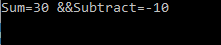#### use of out parameter in c#

Total Post:19

Points:135
520  View(s)
Ratings:
Rate this:
i have little confujion in out keyword, where we use this, please give an example
1.Post:9

Points:63
##### Re: use of out parameter in c#

when you are want to return any value from any method then you are use return keyword. But return  keyword return only one value at a time.
Let see in Example:

```using System; class Program {
public static void Main()   {      int result = 0;      result = Add(10, 20);      Console.WriteLine("Sum={0} ", result);      Console.ReadKey();   }
public static int Add(int FN, int SN)   {     return FN + SN;   } }```

in this example method Add  return a single value.
But at the same time i want to return more than one value from a method then return  not do this operation.
So, that place we use out keyword.
Because out keyword used when you want a method to return more than one value.
Let see in below example
Example: I have a method Calculate  and i want to it return two value first is Sum and second is Subtract of numbers
```using System;class Program{   public static void Main()   {       int Total = 0;       int Subtract= 0;       Calculate(10, 20, out Total, out Subtract);       Console.WriteLine("Sum={0} &&Subtract={1}",Total,Subtract);       Console.ReadKey();   }
public static void Calculate(int FN,int SN,out int Sum,out int Subtract)   {       Sum = FN + SN;       Subtract= FN - SN;   }}```

OUTPUT:I hope you are understand this.